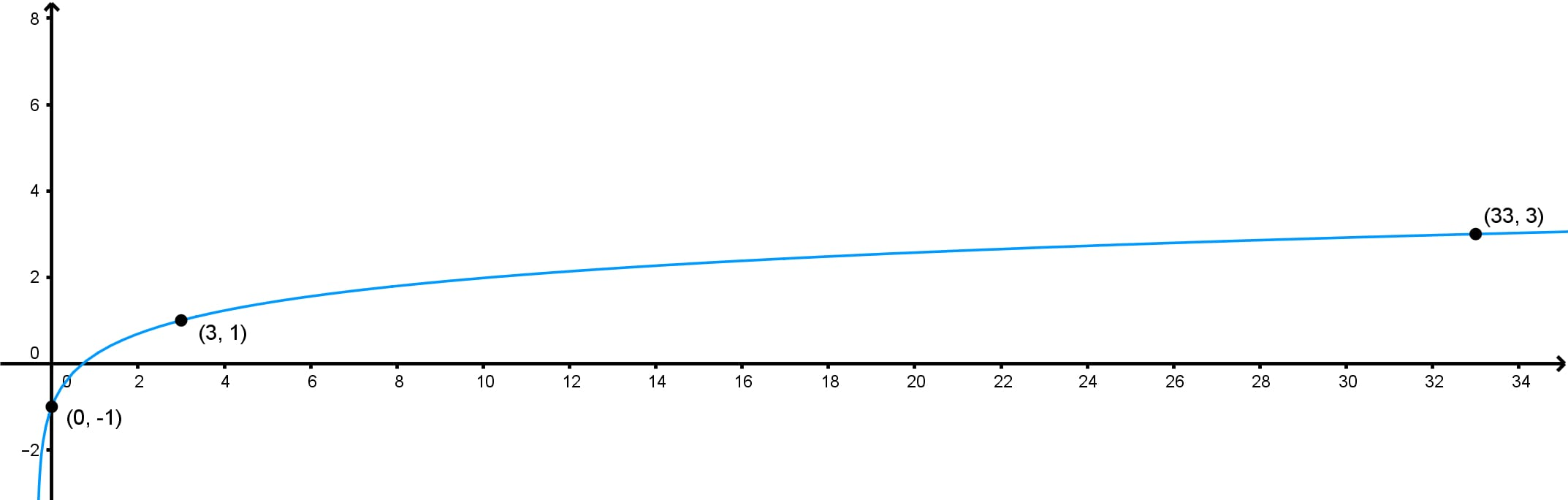# Finding a logarithmic function given its graph

### Finding a logarithmic function given its graph

#### Lessons

• Introduction
Introduction to finding logarithmic function given its graph

i. What is a logarithmic function?

ii. How can we determine the equation of a logarithmic graph?

• 1.
Determining the Equation of an Exponential Function Given Its Graph

Determine an exponential function in the form $y = \log_{b} x$ with the given graph.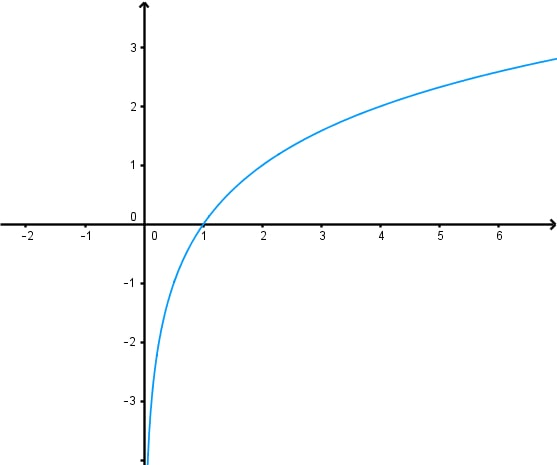• 2.
Determining the Equation of a Transformed Logarithmic function given its Graph

Determine a logarithmic function in the form $y = \log_{2} (x + b) + c$ for each of the given graphs.

a)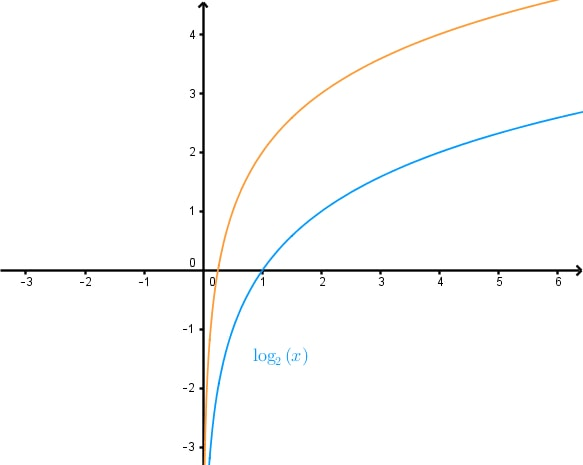b)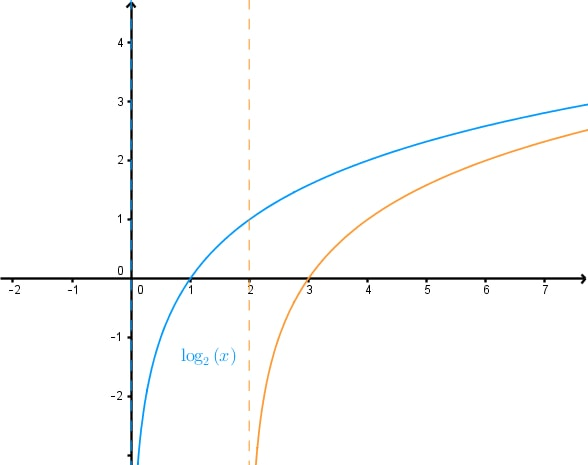• 3.
Determining the Equation of a Transformed Logarithmic function given its Graph - Continued

Determine a logarithmic function in the form $y = A \log_{2} (Bx)$ for each of the given graphs.

a)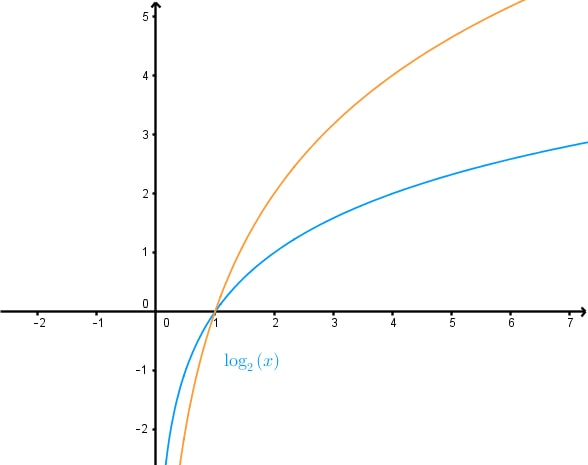b)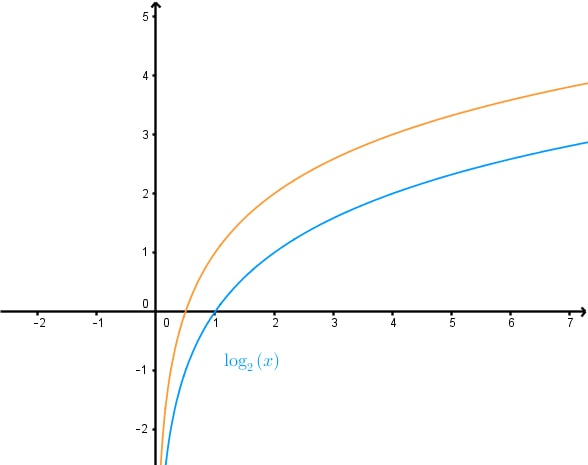c)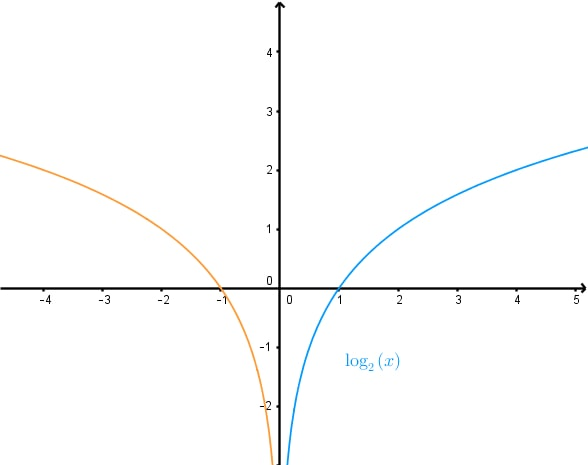d)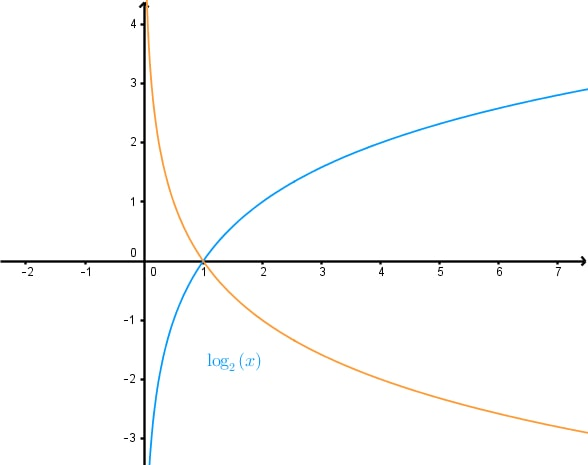• 4.
Determining the Equation of a Logarithmic Graph With Multiple Transformations

Determine a logarithmic function in the form $y = A \log (Bx+1)+C$ for each of the given graphs.